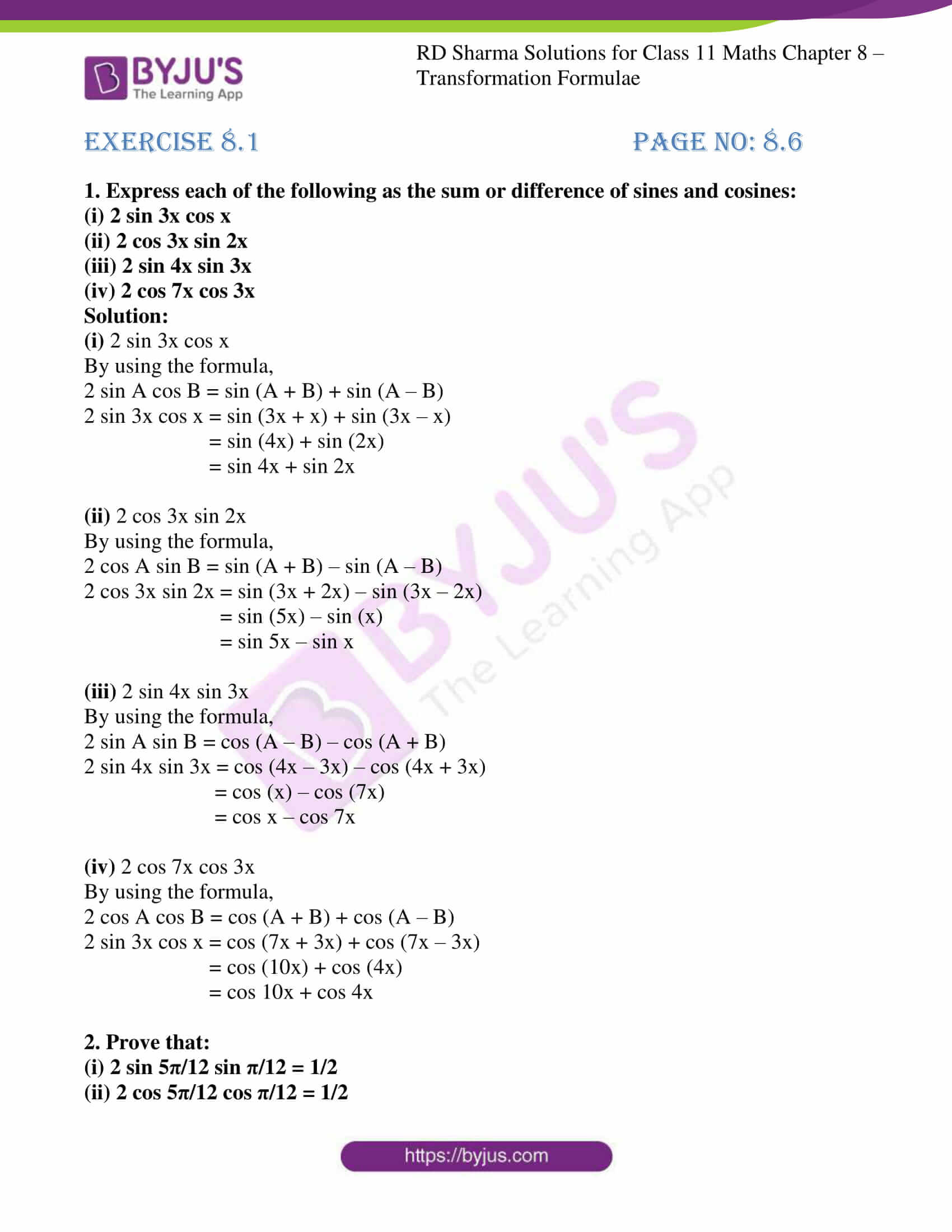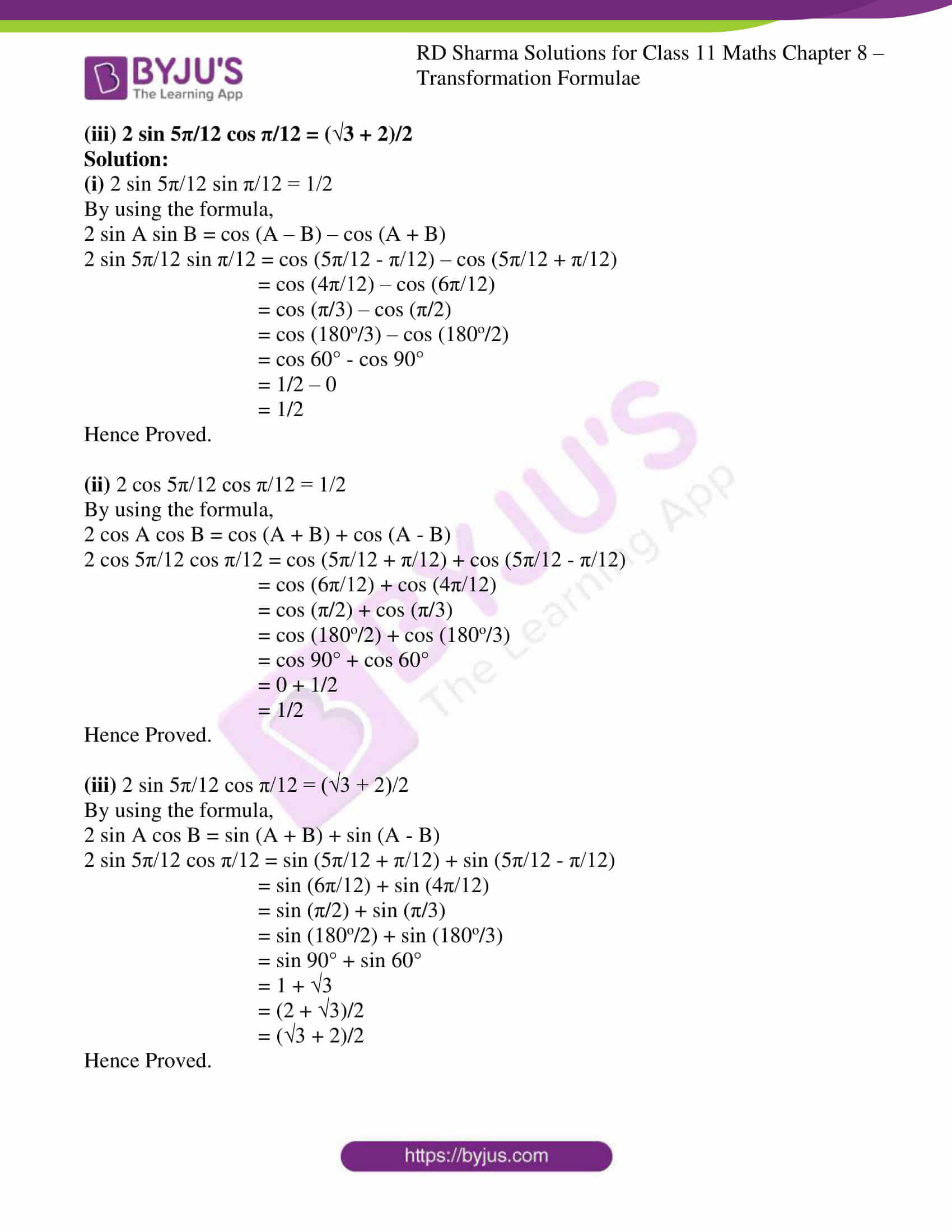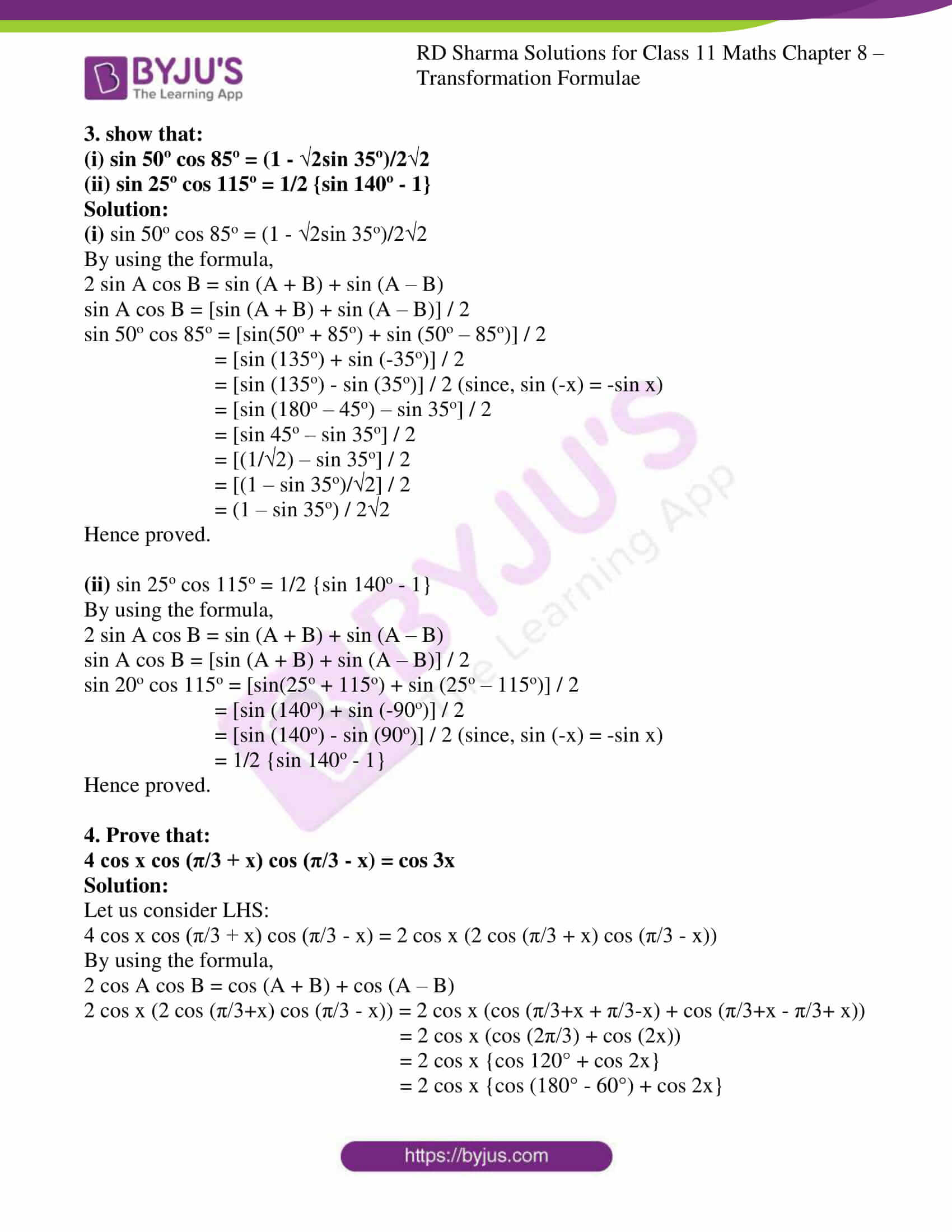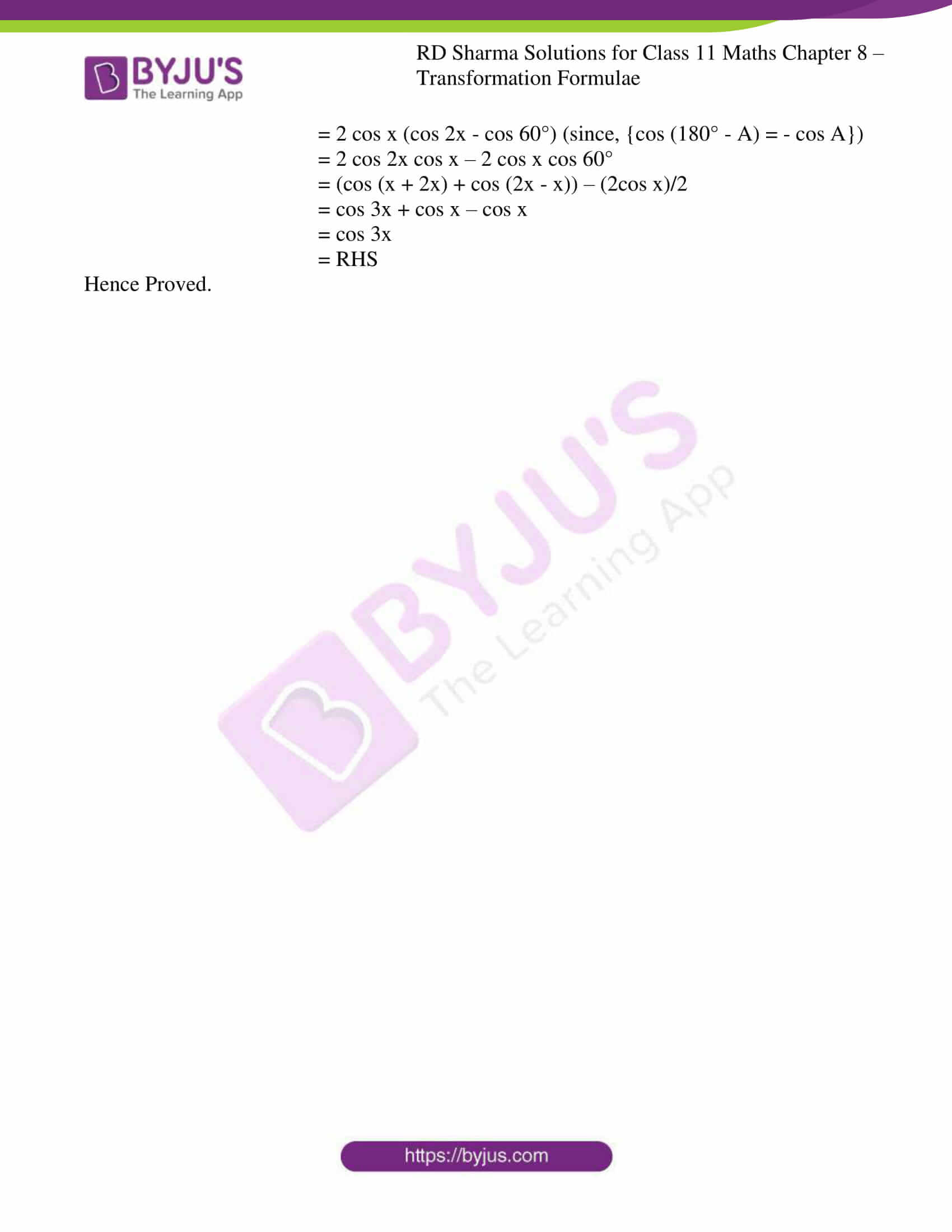# RD Sharma Solutions for Class 11 Chapter 8 - Transformation Formulae Exercise 8.1

In Exercise 8.1 of Chapter 8, we shall discuss problems based on formulae to transform the product into sum or difference. This exercise can be used as a model of reference by the students to improve their conceptual knowledge and understand the different ways used to solve the problems. Students can refer to RD Sharma Class 11 Solutions which are formulated by BYJU’S experts in Maths to increase students confidence, which plays a crucial role in their board exams. The links to the solutions of this exercise can be accessed in the RD Sharma Class 11 Maths pdf, which is available in the below-mentioned links.

## Download the Pdf of RD Sharma Solutions for Class 11 Maths Exercise 8.1 Chapter 8 – Transformation Formulae### Access answers to RD Sharma Solutions for Class 11 Maths Exercise 8.1 Chapter 8 – Transformation Formulae

1. Express each of the following as the sum or difference of sines and cosines:
(i) 2 sin 3x cos x
(ii) 2 cos 3x sin 2x
(iii) 2 sin 4x sin 3x
(iv) 2 cos 7x cos 3x

Solution:

(i) 2 sin 3x cos x

By using the formula,

2 sin A cos B = sin (A + B) + sin (A – B)

2 sin 3x cos x = sin (3x + x) + sin (3x – x)

= sin (4x) + sin (2x)

= sin 4x + sin 2x

(ii) 2 cos 3x sin 2x

By using the formula,

2 cos A sin B = sin (A + B) – sin (A – B)

2 cos 3x sin 2x = sin (3x + 2x) – sin (3x – 2x)

= sin (5x) – sin (x)

= sin 5x – sin x

(iii) 2 sin 4x sin 3x

By using the formula,

2 sin A sin B = cos (A – B) – cos (A + B)

2 sin 4x sin 3x = cos (4x – 3x) – cos (4x + 3x)

= cos (x) – cos (7x)

= cos x – cos 7x

(iv) 2 cos 7x cos 3x

By using the formula,

2 cos A cos B = cos (A + B) + cos (A – B)

2 sin 3x cos x = cos (7x + 3x) + cos (7x – 3x)

= cos (10x) + cos (4x)

= cos 10x + cos 4x

2. Prove that:
(i) 2 sin 5π/12 sin π/12 = 1/2

(ii) 2 cos 5π/12 cos π/12 = 1/2

(iii) 2 sin 5π/12 cos π/12 = (√3 + 2)/2

Solution:

(i) 2 sin 5π/12 sin π/12 = 1/2

By using the formula,

2 sin A sin B = cos (A – B) – cos (A + B)

2 sin 5π/12 sin π/12 = cos (5π/12 – π/12) – cos (5π/12 + π/12)

= cos (4π/12) – cos (6π/12)

= cos (π/3) – cos (π/2)

= cos (180o/3) – cos (180o/2)

= cos 60° – cos 90°

= 1/2 – 0

= 1/2

Hence Proved.

(ii) 2 cos 5π/12 cos π/12 = 1/2

By using the formula,

2 cos A cos B = cos (A + B) + cos (A – B)

2 cos 5π/12 cos π/12 = cos (5π/12 + π/12) + cos (5π/12 – π/12)

= cos (6π/12) + cos (4π/12)

= cos (π/2) + cos (π/3)

= cos (180o/2) + cos (180o/3)

= cos 90° + cos 60°

= 0 + 1/2

= 1/2

Hence Proved.

(iii) 2 sin 5π/12 cos π/12 = (√3 + 2)/2

By using the formula,

2 sin A cos B = sin (A + B) + sin (A – B)

2 sin 5π/12 cos π/12 = sin (5π/12 + π/12) + sin (5π/12 – π/12)

= sin (6π/12) + sin (4π/12)

= sin (π/2) + sin (π/3)

= sin (180o/2) + sin (180o/3)

= sin 90° + sin 60°

= 1 + √3

= (2 + √3)/2

= (√3 + 2)/2

Hence Proved.

3. show that:

(i) sin 50o cos 85o = (1 – √2sin 35o)/2√2

(ii) sin 25o cos 115o = 1/2 {sin 140o – 1}

Solution:

(i) sin 50o cos 85o = (1 – √2sin 35o)/2√2

By using the formula,

2 sin A cos B = sin (A + B) + sin (A – B)

sin A cos B = [sin (A + B) + sin (A – B)] / 2

sin 50o cos 85o = [sin(50o + 85o) + sin (50o – 85o)] / 2

= [sin (135o) + sin (-35o)] / 2

= [sin (135o) – sin (35o)] / 2 (since, sin (-x) = -sin x)

= [sin (180o – 45o) – sin 35o] / 2

= [sin 45o – sin 35o] / 2

= [(1/√2) – sin 35o] / 2

= [(1 – sin 35o)/2] / 2

= (1 – sin 35o) / 22

Hence proved.

(ii) sin 25o cos 115o = 1/2 {sin 140o – 1}

By using the formula,

2 sin A cos B = sin (A + B) + sin (A – B)

sin A cos B = [sin (A + B) + sin (A – B)] / 2

sin 20o cos 115o = [sin(25o + 115o) + sin (25o – 115o)] / 2

= [sin (140o) + sin (-90o)] / 2

= [sin (140o) – sin (90o)] / 2 (since, sin (-x) = -sin x)

= 1/2 {sin 140o – 1}

Hence proved.

4. Prove that:

4 cos x cos (π/3 + x) cos (π/3 – x) = cos 3x

Solution:

Let us consider LHS:

4 cos x cos (π/3 + x) cos (π/3 – x) = 2 cos x (2 cos (π/3 + x) cos (π/3 – x))

By using the formula,

2 cos A cos B = cos (A + B) + cos (A – B)

2 cos x (2 cos (π/3+x) cos (π/3 – x)) = 2 cos x (cos (π/3+x + π/3-x) + cos (π/3+x – π/3+ x))

= 2 cos x (cos (2π/3) + cos (2x))

= 2 cos x {cos 120° + cos 2x}

= 2 cos x {cos (180° – 60°) + cos 2x}

= 2 cos x (cos 2x – cos 60°) (since, {cos (180° – A) = – cos A})

= 2 cos 2x cos x – 2 cos x cos 60°

= (cos (x + 2x) + cos (2x – x)) – (2cos x)/2

= cos 3x + cos x – cos x

= cos 3x

= RHS

Hence Proved.